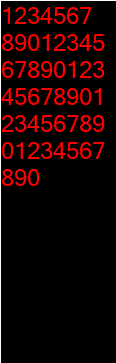# Python在图片中插入大量文字并且自动换行

更新时间：2019年01月02日 15:32:14   作者：staHuri我要评论```from PIL import Image, ImageDraw, ImageFont
class ImgText:
font = ImageFont.truetype("micross.ttf", 24)
def __init__(self, text):
# 预设宽度 可以修改成你需要的图片宽度
self.width = 100
# 文本
self.text = text
# 段落 , 行数, 行高
self.duanluo, self.note_height, self.line_height = self.split_text()
def get_duanluo(self, text):
txt = Image.new('RGBA', (100, 100), (255, 255, 255, 0))
draw = ImageDraw.Draw(txt)
# 所有文字的段落
duanluo = ""
# 宽度总和
sum_width = 0
# 几行
line_count = 1
# 行高
line_height = 0
for char in text:
width, height = draw.textsize(char, ImgText.font)
sum_width += width
if sum_width > self.width: # 超过预设宽度就修改段落 以及当前行数
line_count += 1
sum_width = 0
duanluo += '\n'
duanluo += char
line_height = max(height, line_height)
if not duanluo.endswith('\n'):
duanluo += '\n'
return duanluo, line_height, line_count
def split_text(self):
# 按规定宽度分组
max_line_height, total_lines = 0, 0
allText = []
for text in self.text.split('\n'):
duanluo, line_height, line_count = self.get_duanluo(text)
max_line_height = max(line_height, max_line_height)
total_lines += line_count
allText.append((duanluo, line_count))
line_height = max_line_height
total_height = total_lines * line_height
return allText, total_height, line_height
def draw_text(self):
"""
绘图以及文字
:return:
"""
note_img = Image.open("001.png").convert("RGBA")
draw = ImageDraw.Draw(note_img)
# 左上角开始
x, y = 0, 0
for duanluo, line_count in self.duanluo:
draw.text((x, y), duanluo, fill=(255, 0, 0), font=ImgText.font)
y += self.line_height * line_count
note_img.save("result.png")
if __name__ == '__main__':
n = ImgText(
"1234567890" * 5)
n.draw_text()```